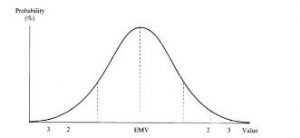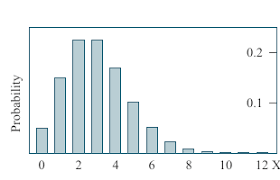## How to Calculate and Solve for Mean | ProbabilityThe image above represents mean.

To compute for mean, two essential parameters are needed and these are number of possible outcomes in any single trial (n) and probability of success in any single trial (p).

The formula for calculating mean:

μ = np

Where;

μ = Mean
n = Number of Possible Outcomes in Any Single Trial
p = Probability of success in any single trial

Let’s solve an example;
Find the mean when the number of possible outcomes in any single trial is 8 and the probability of success in any single trial is 1.

This implies that;

n = Number of Possible Outcomes in Any Single Trial = 8
p = Probability of success in any single trial = 1

μ = np
μ = (8)(1)
μ = 8

Therefore, the mean is 8.

Calculating for the Number of Possible Outcomes in Any Single Trial when the Mean and the Probability of Success in Any Single Trial is Given.

n = μ / p

Where;

n = Number of Possible Outcomes in Any Single Trial
μ = Mean
p = Probability of Success in Any Single Trial

Let’s solve an example;
Find the number of possible outcomes in any single trial when the mean is 15 and the probability of success in any single trial is 1.

This implies that;

μ = Mean = 15
p = Probability of Success in Any Single Trial = 1

n = μ / p
n = 15 / 1
n = 15

Therefore, the number of possible outcomes in any single trial is 15.

## How to Calculate and Solve for Poisson Probability Distribution | ProbabilityThe image above represents Poisson probability distribution.

To compute for Poisson probability distribution, two essential parameters are needed and these parameters are Mean of the Theoretical Distribution (μ) and Number of successes of event A (r).

The formula for Poisson probability distribution:

P(x = r) = e-μ(μr)r!

Where;

P(x = r) = Poisson Probability Distribution
r = Number of Successes of Event A
μ = Mean of the Theoretical Distribution

Let’s solve an example;
Find the poisson probability distribution when the mean of the theoretical distribution is 12 and the number of successes of event A is 6.

This implies that;

r = Number of Successes of Event A = 6
μ = Mean of the Theoretical Distribution = 12

P(x = r) = e-μ(μr)r!
P(x = r) = e-12(12)66!
P(x = r) = (0.00000614)(2985984)(720)
P(x = r) = 0.025

Therefore, the Poisson probability distribution is 0.025.